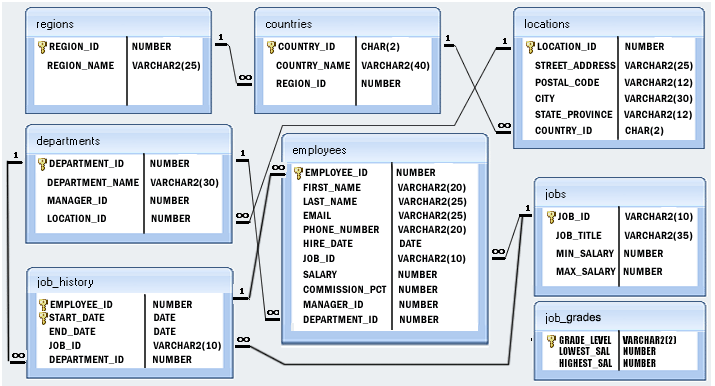﻿ Pandas HR database: Display the name, salary and manger id where manager ids are null - w3resource# Pandas HR database: Display the name, salary and manger id where manager ids are null

## Pandas HR database Queries: Exercise-10 with Solution

Write a Pandas program to display the first name, last name, salary and manger id where manager ids are null.

Sample Solution :

Python Code :

``````import pandas as pd
print("First name      Last name       Salary    Manager ID")
result = employees[employees['manager_id'].isnull()]
for index, row in result.iterrows():
print(row['first_name'].ljust(15),row['last_name'].ljust(15),str(row['salary']).ljust(9),row['manager_id'])
``````

Sample Output:

```First name      Last name       Salary    Manager ID
Steven          King            24000     nan
```

Click to view the table contain:

Employees Table

Departments Table

Countries Table

Job_History Table

Jobs Table

Locations Table

Regions Table

Python Code Editor:

Structure of HR database :Have another way to solve this solution? Contribute your code (and comments) through Disqus.

What is the difficulty level of this exercise?

﻿

## Python: Tips of the Day

Checks if the given number falls within the given range.

Example:

```def tips_range(n, start, end = 0):
return start <= n <= end if end >= start else end <= n <= start
print(tips_range(2, 4, 6))
print(tips_range(4, 8))
print(tips_range(1, 3, 5))
print(tips_range(1, 3))
```

Output:

```False
True
False
True
```